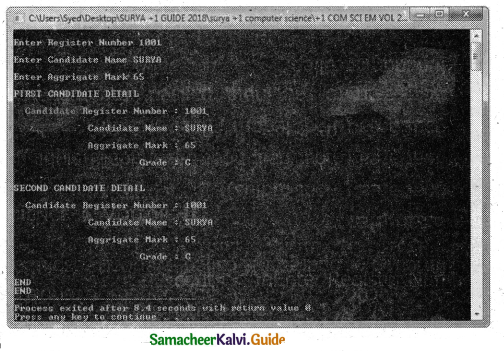Tamilnadu State Board New Syllabus Samacheer Kalvi 11th Computer Science Guide Pdf Chapter 14 Classes and Objects Text Book Back Questions and Answers, Notes.

## Tamilnadu Samacheer Kalvi 11th Computer Science Solutions Chapter 14 Classes and Objects

### 11th Computer Science Guide Classes and Objects Text Book Questions and Answers

Book Evaluation

Part I

Question 1.
The variables declared inside the class are known as data members and the functions are known as
a) data functions
b) inline functions
c) member functions
d) attributes
c) member functions

Question 2.
Which of the following statements about member functions are True or False?
i) A member function can call another member function directly with using the dot operator.
ii) Member function can access the private data of the class.
a) i-True, ii-True
b) i-False, ii-True
c) i-True, ii-False
d) i-False, ii-False
b) i-False, ii-TrueQuestion 3.
A member function can call another member function directly, without using the dot operator called as
a) sub function
b) sub member
c) nesting of member function
d) sibling of member function
c) nesting of member function

Question 4.
The member function defined within the class behave like
a) inline functions
b) Non inline function
c) Outline function
d) Data function
a) inline functions

Question 5.
Which of the following access specifier protects data from inadvertent modifications?
a) Private
b) Protected
c) Public
d) Global
a) PrivateQuestion 6.
class x
{
inty;
public:
x(int z)
{
y=z;
}
} x1;
intmain( )
{
x x2(10);
return 0;
}
How many objects are created for the above program?
a) 10
b) 14
c) 5
d) 2
c) 5

Question 7.
State whether the following statements about the constructor are True or False.
i) constructors should be declared in the private section.
ii) constructors are invoked automatically when the objects are created.
a) True, True
b) True, False
c) False, True
d) False, False
c) False, TrueQuestion 8.
Which of the following constructor is executed for the following prototype ?
a) Default constructor
b) Parameterized constructor
c) Copy constructor
d) Non Parameterized constructor
c) Copy constructor

Question 9.
What happens when a class with parameterized constructors and having no default constructor is used in a program and we create an object that needs a zero- argument constructor?
a) Compile-time error
b) Domain error
c) Runtime error
d) Runtime exception
a) Compile-time error

Question 10.
Which of the following create a temporary instance?
a) Implicit call to the constructor
b) Explicit call to the constructor
c) Implicit call to the destructor
d) Explicit call to the destructor
b) Explicit call to the constructorPart – II

Question 1.
What are called members?
The class comprises members. Members are classified as Data Members and Member functions. Data members are the data variables that represent the features or properties of a class. Member functions are the functions that perform specific tasks in a class.

Question 2.
Differentiate structure and class though both are user-defined data types.
The only difference between structure and class is the members of the structure are by default public whereas it is private in class.

Question 3.
What is the difference between the class and object in terms of oop?
Object:

• Object is an instance of a class.
• Object is a real-world entity such as pen, laptop, mobile, chair, etc.
• Object allocates memory when it is created.

Class:

• Class is a blueprint or template from which objects are created.
• Class is a group of similar objects.
• Class doesn’t allocate memory when it is created.

Question 4.
Why it is considered a good practice to define a constructor though a compiler can automatically generate a constructor?
A user-defined constructor is the best method of initialise array of objects and normal objects.Question 5.
Write down the importance of the destructor.
The purpose of the destructor is to free the resources that the object may have acquired during its lifetime. A destructor function removes the memory of an object which was allocated by the constructor at the time of creating an object.

Part – III

Question 1.
Rewrite the following program after removing the syntax errors if any and underline the errors:
#include<iostream>
#include<stdio.h>
classmystud
{ intstudid =1001;
char name;
public
mystud( )
{ }
void register ( ) {cin>>stdid;gets(name);
}
void display ( )
{ cout<<studid<<“: “<<name<<endl;}
}
int main( )
{ mystud MS;
register.MS( );
MS.display( );
}
MODIFIED PROGRAM:
#include<iostream>
#include<stdio.h>
class mystud
{
int studid;
char name;
public:
mystud( )
{
studid=1001;
}
void register ( )
{
cin>>stdid;
gets(name);
}
void display ( )
{
cout<<studid<<“: “<<name<<endl;
}
};
int main( )
{
mystud MS;
MS.reqister( );
MS.display( );
}Question 2.
Write with an example of how will you dynamically initialize objects?
Dynamic initialization of Objects:
When the initial values are provided during runtime then it is called dynamic initialization.
Program to illustrate dynamic initialization
#include
using namespace std;
class X
{
int n;
float avg;
public:
X(int p,float q)
{
n=p;
avg=q;
}
void disp( )
{
cout<<“\n Roll numbe:-” <<n;
cout<<“\nAverage :-“<<avg;
}
};
int main( )
{
int a ; float b;
cout<<“\nEnter the Roll Number”;
cin>>a; .
cout<<“\nEnter the Average”;
cin>>b;
X x(a,b); // dynamic initialization
x.disp( );
return 0;
}
Output
Enter the Roll Number 1201
Enter the Average 98.6
Roll number:- 1201
Average :- 98.6Question 3.
What are advantages of declaring constructors and destructor under public access ability?

When constructor and destructor are declared under public:

1. we can initialize the object while declaring it.
2. we can explicitly call the constructor.
3. we can overload constructors and therefore use multiple constructors to initialize objects automatically.
4. we can destroy the objects at the end of class scope automatically (free unused memory).

However, some C++ compiler and Dev C++ do not allow to declare constructor and destructor under private section. So it is better to declare constructor and destructor under public section only.

Question 4.
Given the following C++ code, answer the questions (i) & (ii).
class TestMeOut
{
public:
~TestMeOut( ) //Function 1
{
cout<<“Leaving the examination hall”<<endl;
}
TestMeOut( ) //Function 2
{
cout<<“Appearing for examination'<<endl;
}
void MyWork( ) //Function 3
{
cout<<“Attempting Questions//<<endl;
}
};
i) In Object-Oriented Programming, what is Function 1 referred to as and when does it get invoked/called?
Function 1 is called a destructor. It will be automatically invoked when the object goes out of scope (ie. at the end of a program).

ii) In Object-Oriented Programming, what is Function 2 referred to as, and when does it get invoked/called?
Function2 is called a constructor. It will be automatically invoked when an object comes into scope.(ie. at the time of object creation).Question 5.
Write the output of the following C++ program code:
#include<iostream>
using namespace std;
class Calci
{
int Bonus;
public:
Calci( )
{
Bonus=0;
}//ascii value of A=65
void Down(int G)
{
}
void Up(int G)
{
Bonus++;
}
void Show( )
{
}
};
int main( )
{
Calci c;
c.Down(3);
c.Show( );
c.Up(7);
c.Show( );
c.Down(2);
c.Show( );
return 0;
}
Output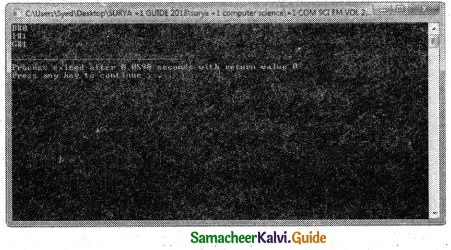Part – IV

Explain In Detail

Question 1.
Explain nested class with example.
When one class becomes a member of another class then it is called Nested class and the relationship is called containership. When a class is declared within another class, the inner class is called a Nested class (i.e. the inner class) and the outer class is known as the Enclosing class. The nested class can be defined in private as well as in the public section of the Enclosing class.

Classes can be nested in two ways:

1. By defining a class within another class
2. By declaring an object of a class as a member to another class
3. By defining a class within another class

C++ program to illustrate the nested class
#include<iostream>
using namespace std;
class enclose
{
private:
int x;
class nest
{
private :
int y;
public:
int z;
void prn( )
{
y=3;z=2;
cout<<“\n The product of”
< <y< <‘*'< <z<<“= “< <y*z< <“\n”;
}
}; //inner class definition over
nest m1;
public:
nest n2;
void square( )
{
n2.prn( ); //inner class member function is called by its object
x=2;
n2.z=4;
cout<<“\n The product of” <<n2.z<<‘*'<<n2.z<<“=”n2.z*n2.z<<“/n”;
cout<<“\n The product of” <<x<<‘*'<<x<<“= “<<x*x;
}
}; //outer class definition over
int main( )
{
enclose e;
e.square( ); //outer class member function is called
}
Output
The product of 3*2=6
The product of 4*4=16
The product of 2*2=4

In the above program the inner class nest is defined inside the outer class enclose, nest is accessed by enclose by creating an object of nestQuestion 2.
Mention the differences between constructor and destructor.

 CONSTRUCTOR DESTRUCTOR The name of the constructor must be same as that of the class. The destructor has the same name as that of the class prefixed by the tilde character A constructor can have parameter list. The destructor cannot have arguments. The constructor function can be overloaded. Destructors cannot be overloaded i.e., there can be only one destructor in a class. Constructor cannot be inherited but a derived class can call the base class constructor. Destructor cannot be inherited. The constructor is executed automatically when the object is created. The destructor is executed automatically when the control reaches the end of class. Allocated memory space for the object. Destroy the object.

Question 3.
Define a class RESORT with the following description in C++ :
Private members:
Rno // Data member to storeroom number
Name //Data member to store user name
Charges //Data member to store per day charge
Days //Data member to store the number of days
Compute ( ) // A function to calculate total amount as Days * Charges and if the
//total amount exceeds 11000 then total amount is 1.02 * Days *Charges

Public member:
getinfo( ) // Function to Read the information like name , room no, charges and days
dispinfo ( ) // Function to display all entered details and total amount calculated
//using COMPUTE function
PROGRAM
using namespace std;
#include<iostream>
class RESORT
{
private:
int Rno,Days,Charges;
char Rname;
int compute( )
{
if (Days * Charges >11000)
return (Days * Charges * 1.02);
else
return(Days * Charges);
}
public:
getinfo( )
{
cout<<“\nEnter customer name : “;
cin>>Rname;
cout<<‘nEnter charges per day : “;
cin>>Charges;
cout< <‘nEnter Number of days : “;
cin>>Days;
cout<<‘n Enter Room Number : “;
cin>>Rno;
}
dispinfo( )
{
cout<<‘nRoom Number :
“<<Rno;
cout<<‘nCustomer name :
“<<Rname;
cout<<‘nCharges per day :
“<<Charges;
cout<<‘nNumber of days :
“<<Days;
cout<<‘nTotal Amount :
“<<compute( );
}
int main( )
{
RESORT Obj;
Obj,getinfo( );
Obj.dispinfo( );
Output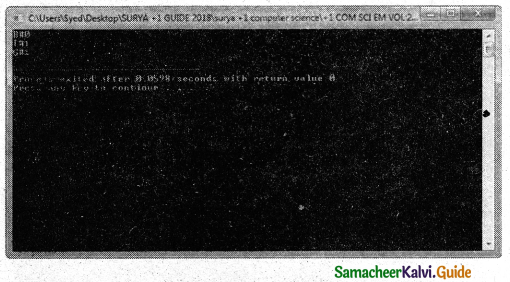Question 4.
WrIte the output of the following:
#include<iostream>
#indude<stdio.h>
using namespace std;
class sub
{
int day, subno;
public :
sub(int,int); // prototype
void printsub( )
{
cout<<” subject number: “<<subno;
cout<<” Days : ” <<day;
}
};
sub::sub(int d=150,int sn=12)
{
cout<<endl<<“Constructing the object
“<<endl;
day=d;
sub no=sn;
}
class stud ‘
{
int rno;
float marks; public:
stud( )
{
cout<< “Constructing the object of
students “<<endl;
rno=0;
marks=0.0;
}
void getval( )
{
cout<<“Enter the roll number and the marks secured”; cin>>rno>>marks;
}
void printdet( )
{
cout<<“Roll no : “<<rno<<“Marks : “<<marks<<endl; .
}
};
{
sub obj;
stud objone;
float fees; ,
public :
{
cout<< “Constructing the object of admission “<<endl;
fees=0.0;
}
void printdet( )
{
objone.printdet( );
obj.printsub();
cout<<“fees : “<<fees<<endl;
}
};
int main( )
{
system (“cls”);
cout<<endl<< ?’Back in main ()”;
return 0;
}
Output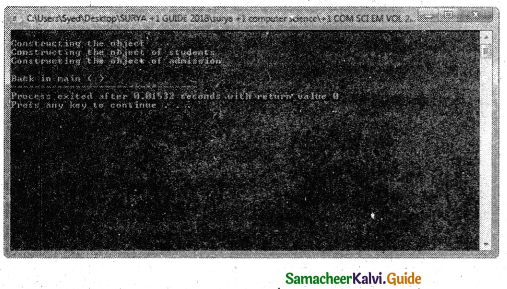Question 5.
Write the output of the following.
#indude<iostream>
#include<stdio,h>
using namespace std;
class P
{
public:
P( )
{
cout<< “\nConstructor of class P }
~P( )
{
cout< < “\nDestructor of class P
}
};
class Q
{
public:
Q( )
{
cout< <“\nConstructor of class Q “;}
~ Q( )
{
cout<< “\nDestructor of class Q
}
};
class R
{
P obj1, obj2;
Q obj3;
public:
R( )
{
cout<< “\nConstructor of class R “;}
~R( )
{
cout<< “\nDestructor of class R
}
};
int main ( )
{
R oR; :
Q oq;
Pop;
return 0;
Output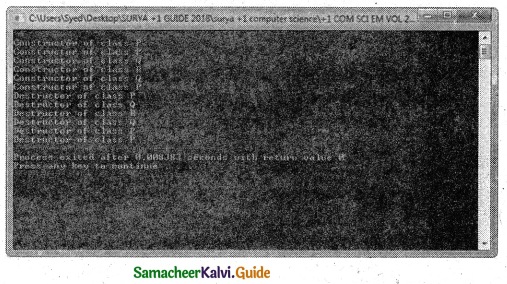### 11th Computer Science Guide Classes and Objects Additional Questions and Answers

Choose The Correct Answer (1 Mark)

Question 1.
The most important feature of C++ is ………………..
(a) object
(b) class
(c) public
(d) All the above
(b) class

Question 2.
How many features are commonly present in OOP languages?
a) 3
b) 2
c) 4
d) 5
c) 4

Question 3.
Calling a member function of an object is also known as ……………….. to object.
(a) call function
(b) call by value
(c) call by reference
(d) sending message
(d) sending messageQuestion 4.
……………… is a way to bind the data and its associated functions together,
a) Class
b) Array
c) Structure
d) All the above
a) Class

Question 5.
When one class become a member of another class, the relationship is called ………………..
(a) containership
(b) partnership
(c) friendship
(d) all the above
(a) containership

Question 6.
The body of the class is defined inside the ………………. brackets.
a) Angle < >
b) Square [ ]
c) Curly { }
d) None of these
c) Curly { }Question 7.
……………….. can be defined either in private or in the public section of a class.
(a) Object
(b) Data type
(c) Memory
(d) constructor
(d) constructor

Question 8.
The members of the structure are by default ………………..
a) Private
b) Public
c) Protected
d) None of these
b) Public

Question 9.
There are ……………….. ways to create an object using the parameterized constructor.
(a) 3
(b) 2
(c) 1
(d) 4
(c) 1

Question 10.
The class body contains ………………….
a) Data members
b) Member functions
c) Both A and B
d) None of these
c) Both A and BQuestion 11.
The class body has………………… access specifiers.
a) Three
b) Four
c) Two
d) Five
a) Three

Question 12.
The class body has…………….. access specifiers.
a) Private
b) Public
c) Protected
d) All the above
d) All the above

Question 13.
…………………. is a visibility label.
a) Private
b) Public
c) Protected
d) All the above
d) All the above

Question 14.
……………….. allows preventing the functions of a program to access directly the internal representation of a class type.
a) Data Hiding
b) Data Capturing
c) Data Processing
d) None of these
a) Data HidingQuestion 15.
The access restriction to the class members is specified by ……………. section within the class
body.
a) Private
b) Public
c) Protected
d) All the above
d) All the above

Question 16.
A …………………. member is accessible from where outside the class but within a program.
a) Private
b) Public
c) Protected
d) All the above
b) Public

Question 17.
We can set and get the value of public data members using ………………… function.
a) Member
b) Nonmember
c) Either A or B
d) None of these
c) Either A or BQuestion 18.
A …………….. member cannot be accessed from outside the class.
a) Private
b) Public
c) Protected
d) All the above
a) Private

Question 19.
Only the class member functions can access ……………… members.
a) Private
b) Public
c) Protected
d) All the above
a) Private

Question 20.
……………….. members can be accessed in child classes.
a) Private
b) Public
c) Protected
d) All the above
c) ProtectedQuestion 21.
If all members of the class are defined as …………….. then the class become frozen.
a) Private
b) Public
c) Protected
d) All the above
a) Private

Question 22.
If all members of the class are defined as ………………….. then the object of the class can not access anything from the class,
a) Private
b) Public
c) Protected
d) All the above
a) Private

Question 23.
………………. are the data variables that represent the features or properties of a class.
a) Data members
b) Member functions
c) Both A and B
d) None of these
a) Data members

Question 24.
………………… are the functions that perform specific tasks in a class.
a) Data members
b) Member functions
c) Both A and B
d) None of these
b) Member functionsQuestion 25.
Member functions are called as …………………..
a) Methods
b) Attributes
c) Properties
d) None of these
a) Methods

Question 26.
Data members are also called as ……………….
a) Methods
b) Attributes
c) Properties
d) None of these
b) Attributes

Question 27.
Classes contain a special member function called as ……………………
a) Constructors
b) Destructors
c) Both A and B
d) None of these
c) Both A and BQuestion 28.
The member functions of a class can be defined in ……………….. ways.
a) Two
b) Three
c) Four
d) Five
a) Two

Question 29.
The member functions of a class can be defined in ………………. way.
a) Inside the class definition
b) Outside the class definition
c) Either A or B
d) None of these
c) Either A or B

Question 30.
When a member function is defined Inside a class, it behaves like ………………. functions.
a) Inline
b) General
c) Local
d) None of these
a) InlineQuestion 31.
If a function is inline, the compiler places a copy of the code of that function at each point where the function is called at ………………….
a) Run Time
b) Compile time
c) Both A and B
d) None of these
b) Compile time

Question 32.
When Member function defined outside the class, and then it is be called as ………………….
member function.
a) Outline
b) Non-inline
c) Either A or B
d) None of these
c) Either A or B

Question 33.
When Member function defined outside the class using …………….. operator.
a) Scope resolution
b) Membership
c) Reference
d) Conditional
a) Scope resolutionQuestion 34.
The class variables are called ………………….
a) Object
b) Attributes
c) Procedures
d) None of these
a) Object

Question 35.
Objects are also called as …………………. of class.
a) Instant
b) Instance
c) Attributes
d) None of these
b) Instance

Question 36.
Objects can be created in ………………… methods.
a) Three
b) Four
c) Two
d) Five
c) TwoQuestion 37.
Objects can be created as ……………….
a) Global object
b) Local object
c) Either A or B
d) None of these
c) Either A or B

Question 38.
…………….. objects can be used by any function in the program.
a) Global object
b) Local object
c) Either A or B
d) None of these
a) Global object

Question 39.
If an object is declared outside all the function bodies or by placing their names immediately after the closing brace of the class declaration then it is called as ……………….
a) Global object
b) Local object
c) Either A or B
d) None of these
a) Global objectQuestion 40.
If an object is declared within a function then it is called ……………….
a) Global object
b) Local object
c) Either A or B
d) None of these
b) Local object

Question 41.
……………….. object can not be accessed from outside the function.
a) Global
b) Local
c) Either A or B
d) None of these
b) Local

Question 42.
No separate space is allocated for …………………. when the objects are created.
a) Member functions
b) Data members
c) Both A and B
d) None of these
a) Member functionsQuestion 43.
Memory space required for the ……………….
a) Member functions
b) Data members
c) Both A and B
d) None of these
b) Data members

Question 44.
The members of a class are referenced (accessed) by using the object of the class followed by the ………………. operator.
a) Scope resolution
b) Conditional
c) Dot (membership)
d) None of these
c) Dot (membership)

Question 45.
Calling a member function of an object is also known as ……………….
a) Sending a message to object
b) Communication with the object
c) Either A or B
d) None of these
c) Either A or BQuestion 46.
An array which contains the class type of element is called …………………
a) Array of objects
b) Structure Objects
c) Block of objects
d) None of these
a) Array of objects

Question 47.
The ………………… of the outline member function given in a class specification, instructs the compiler about its visibility mode.
a) Name
b) Prototype
C) Data type
d) None of these
b) Prototype

Question 48.
A member function can call another member function of the same class directly without using the dot operator is called ………………………
a) Nesting of the member function
b) Invariant Members
c) Variant Members
d) None of these
a) Nesting of the member functionQuestion 49.
A member function can call another member function of the same class for that you do not
need a(n) …………………..
a) Member function
b) Data Member
c) Object
d) None of these
c) Object

Question 50.
A member function can access ………………. functions.
a) Public
b) Private
c) Both A and B
d) None of these
c) Both A and B

Question 51.
…………………. operator will reveal the hidden file scope(global) variable.
a) Membership
b) Conditional
c) Scope resolution
d) All the above
c) Scope resolutionQuestion 52.
When an object is passed by ……………… the function creates its own copy of the object and works on it.
a) Value
b) Reference
c) Either A or B
d) None of these
a) Value

Question 53.
When an object is passed by …………….. changes made to the object inside the function do not affect the original object.
a) Value
b) Reference
c) Either A or B
d) None of these
a) Value

Question 54.
When an object is passed by ……………….. its memory address is passed to the function so the called function works directly on the original object used in the function call.
a) Value
b) Reference
c) Either A or B
d) None of these
b) ReferenceQuestion 55.
When an object is passed by ………………………. any changes made to the object inside the function definition are reflected in the original object.
a) Value
b) Reference
c) Either A or B
d) None of these
b) Reference

Question 56.
Member Functions can ………………….
a) Receive object as an argument
b) Return an object
c) Both A and B
d) None of these
c) Both A and B

Question 57.
When one class become a member of another class then it is called ……………. class.
a) Nested
b) Inline
c) External
d) Global
a) NestedQuestion 58.
When one class becomes a member of another class then the relationship is called ………………….
a) Containership
b) Nesting
c) Parent-Child
d) None of these
a) Containership

Question 59.
Classes can be nested in ……………….. ways.
a) Three
b) Two
c) Four
d) Five
b) Two
Question 60.
Classes can be nested in ………………..way.
a) By defining a class within another class
b) By declaring an object of a class as a member to another class
c) Either A or B
d) None of these
c) Either A or BQuestion 61.
When a class is declared within another class, the inner class is called a Nested class (ie the inner class) and the outer class is known as …………………… class.
a) Enclosing
b) Abstract
c) Transit
d) None of these
a) Enclosing

Question 62.
The nested class can be defined in ………………….. section of the Enclosing class.
a) Private
b) Public
c) Either Private or Public
d) None of these
c) Either Private or Public

Question 63.
Whenever an object of a class is declared as a member of another class it is known as a _____ class.
a) Abstract
b) Container
c) Literal
d) None of these
b) ContainerQuestion 64.
Instantiating object is done using ……………….
a) Constructor
b) Destructor
c) Data abstraction
d) Data hiding
a) Constructor

Question 65.
A(n) ………………..in C++ can be initialized during the time of their declaration.
a) Array
b) Structure
c) Array or Structure
d) None of these
c) Array or Structure

Question 66.
Member function of a class can access all the members irrespective of their associated …………………..
a) Access specifier
b) Data type
c) Return type
d) Argument
a) Access specifierQuestion 67.
When an instance of a class comes into scope, a special function called the ……………. gets executed.
a) Constructor
b) Destructor
c) Data abstraction
d) Data hiding
a) Constructor

Question 68.
The constructor function name has the same name as the …………….. name.
a) Object
b) Class
c) Data member
d) None of these
b) Class

Question 69.
The constructors return ………………
a) int
b) char
c) float
d) nothing
d) nothingQuestion 70.
…………………. are not associated with any data type
a) Constructor
b) Data member
c) Data abstraction
d) Member functions
a) Constructor

Question 71.
……………… can be defined either inside class definition or outside the Class definition.
a) Constructor
b) Destructor
c) Data abstraction
d) Member functions
a) Constructor

Question 72.
A constructor can be defined in ……………… section of a class.
a) Private
b) Public
c) Either Private or Public
d) None of these
c) Either Private or PublicQuestion 73.
If a constructor is defined in ………………… section of a class, then only its object Can be created in any function.
a) Private
b) Public
c) Either Private or Public
d) None of these
b) Public

Question 74.
The main function of the constructor is ……………………….
a) To allocate memory space to the object
b) To initialize the data member of the class object
c) Either A or B
d) None of these
c) Either A or B

Question 75.
A constructor that accepts no parameter is called …………………… constructor.
a) Null
b) Default
c) Empty
d) None of these
b) DefaultQuestion 76.
Identify the correct statement from following with respect to constructor.
a) If a class does not contain an explicit constructor (user defined constructor) the compiler automatically generate a default constructor implicitly as an inline public member.
b) In the absence of user defined constructor the compiler automatically provides the default constructor. It simply allocates memory for the object.
c) Parameterized constructor is achieved by passing parameters to the function.
d) All the above
d) All the above

Question 77.
A constructor which can take arguments is called ……………….. constructor.
a) Parmeterized
b) Default
c) Empty
d) None of these
a) Parmeterized

Question 78.
……………….. type of constructor helps to create objects with different initial values.
a) Parmeterized
b) Default
c) Empty
d) None of these
a) ParmeterizedQuestion 79.
Declaring a constructor with arguments hides the ……………………
a) Data members
b) Compiler generated constructor
c) Member functions
d) None of these
b) Compiler generated constructor

Question 80.
………………… Constructor is used to creating an array of objects.
a) Default
b) Parameterized
d) None of these
a) Default

Question 81.
There are ………………. ways to create an object using the parameterized constructor.
a) Three
b) Two
c) Four
d) Five
b) TwoQuestion 82.
……………… is a way to create an object using the parameterized constructor,
a) Implicit call
b) Explicit call
c) Either A or B
d) None of these
c) Either A or B

Question 83.
In …………….. method, the parameterized constructor is invoked automatically
whenever an object is created.
a) Implicit call
b) Explicit call
c) Either A or B
d) None of these
a) Implicit call

Question 84.
In the………………….. method, the name of the constructor is explicitly given to invoking the parameterized constructor.
a) Implicit call
b) Explicit call
c) Either A or B
d) None of these
b) Explicit callQuestion 85.
………………… method is the most suitable method as it creates a temporary object
a) Implicit call
b) Explicit call
c) Either A or B
d) None of these
b) Explicit call

Question 86.
The chance of data loss will not arise in ………………….. method.
a) Implicit call
b) Explicit call
c) Either A or B
d) None of these
b) Explicit call

Question 87.
A ……………….. object lives in memory as long as it is being used in an expression.
a) Temporary
b) Nested
c) Inline
d) None of these
a) TemporaryQuestion 88.
A constructor having a reference to an already existing object of its own class is called ………………….. constructor.
a) Reference
b) Value
c) Copy
d) Move
c) Copy

Question 89.
A copy constructor is called ……………..
a) When an object is passed as a parameter to any of the member functions
b) When a member function returns an object
c) When an object is passed by reference to an instance of its own class
d) All the above
d) All the above

Question 90.
The constructors are executed in the …………………. of the object declared.
a) Order
b) Reverse order
c) Either A or B
d) None of these
a) OrderQuestion 91.
When the initial values are provided during runtime then it is called ………………….. initialization.
a) Static
b) Dynamic
c) Run time
d) None of these
b) Dynamic

Question 92.
…………………. constructor can have parameter list.
a) Constructor
b) Destructor
c) Both A and B
d) None of these
a) Constructor

Question 93.
No return type can be specified for ………………….
a) Constructor
b) Destructor
c) Both A and B
d) None of these
c) Both A and BQuestion 94.
The ……………….. function can be overloaded.
a) Constructor
b) Destructor
c) Both A and B
d) None of these
a) Constructor

Question 95.
The compiler generates a ………………… in the absence of a user-defined.
a) Constructor
b) Destructor
c) Both A and B
d) None of these
a) Constructor

Question 96.
Compiler generated constructor is ………………….. member function.
a) private
b) protected
c) public
d) None of these
c) publicQuestion 97.
The ………………. is executed automatically,
a) Constructor
b) Destructor
c) Both A and B
d) None of these
c) Both A and B

Question 98.
The ……………. is executed automatically when the object is created.
a) Constructor
b) Destructor
c) Both A and B
d) None of these
a) Constructor

Question 99.
When a class object goes out of scope, a special function called the ………………. gets executed.
a) Constructor
b) Destructor
c) Both A and B
d) None of these
b) DestructorQuestion 100.
The destructor has the same name as the class tag but prefixed with a …………………
a) ~ (tilde)
b) #
c) @
d) None of these
a) ~ (tilde)

Question 101.
A …………………… is a special member function that is called when the lifetime of an object ends.
a) Constructor
b) Destructor
c) Both A and B
d) None of these
b) Destructor

Question 102.
The ………………… cannot have arguments,
a) Constructor
b) Destructor
c) Both A and B
d) None of these
b) DestructorQuestion 103.
There can be ……………… destructor in a class.
a) Two
b) Three
c) Only one
d) Four
c) Only one

Question 104.
_____ cannot be inherited.
a) Constructor
b) Destructor
c) Both A and B
d) None of these
c) Both A and B

Question 1.
Define methods of a class and write its types.
The class comprises members. Member functions are called methods. The member functions of a class can be defined in two ways.

1. Inside the class definition
2. Outside the class definition

Question 2.
Why classes are needed?
Classes are needed to represent real-world entities that not only have data type properties but also have associated operations. It is used to create user-defined data types.Question 3.
What is called as nesting of member functions?
Only the public members of a class can be accessed by the object of that class, using the dot operator. However, a member function can call another member function of the same class directly without using the dot operator. This is called as nesting of member functions.

Question 4.
What are the visibility labels of a class body?
The class body has three visibility labels viz., private, public, and protected. The Visibility labels are also called as access specifiers.

Question 5.
What is a parameterized constructor?
A constructor which can take arguments is called a parameterized constructor. This type of constructor helps to create objects with different initial values. This is achieved by passing parameters to the function.

Question 6.
What happened if all the members of a class are defined as private?
If all members of the class are defined as private, then the class becomes frozen.
The object of the class can not access anything from the class.Question 7.
A class specification just defines the properties of a class. To make use of a class specified, the variables of that class type have to be declared. The class variables are called objects. Objects are also called an instance of the class.

For example:
student s;
In the above statement ‘s’ is an instance of the class student.

Question 8.
How many ways objects can be created for a class? Give its types.
Objects can be created in two methods:

1. Global object
2. Local object

Question 9.
What do you mean by an array of objects?
An array which contains the class type of element is called an array of objects. It is declared and defined in the same way as any other type of array.

Example:
class stock
{
int itemno;
float price; public:
}s;
Here s is an array of objects.Question 10.
What is a nested member function?
A member function can cal! another member function of the same class directly without using the dot operator. This is called as nesting of member functions.

Question 11.
How many ways objects can be passed to function argument?
Objects can also be passed in both ways

• Pass By Value
• Pass By Reference

Question 12.
What is a container class?
Whenever an object of a class is declared as a member of another class it is known as a container class. In the container-ship, the object of one class is declared in another class.

Question 13.
What is the need for a constructor in a class?
Instantiating object is done using constructor. An array or a structure in C++ can be initialized during the time of their declaration using constructor. The constructor function initializes the class object.Question 14.
What are the functions of a constructor?
The main functions of the constructor are:

• To allocate memory space to the object and
• To initialize the data member of the class object.

Question 15.
What is a default constructor?
Default constructor:
A constructor that accepts no parameter is called the default constructor.
For example in the class Data program Data::Data( ) is the default constructor.
Using this constructor objects are created similar to the way the variables of other data types are created.

Example:
int num; //ordinary variable declaration
Data d1; // object declaration
If a class does not contain an explicit constructor the compiler automatically generates a default constructor implicitly as an inline public member.

Question 16.
What is the significance of default constructor?
Default constructors are very useful to crate objects without having specific initial value. It is also used to create array of objects.

Question 17.
How many ways a constructor can be invoked?
There are two ways to create an object using parameterized constructor:

1. Implicit call
2. Explicit callQuestion 18.
What is copy constructor?
A constructor having a reference to an already existing object of its own class is called copy constructor.
In other words Copy Constructor is a type of constructor which is used to create a copy of an already existing object of a class type.

Question 19.
What is the order of constructor invocation?
The constructors are executed in the order of the object declared. (If it is in same statement left to right)

For example:
Test t1;
Test t2; // the order of constructor execution is first for t1 and then for t2.
Consider the following example
Sample s1,s2,s3 ; //The order of construction is s1 then s2 and finally s3

Question 20.
What do you mean by dynamic initialization of Object?
When the initial values are provided during runtime then it is called dynamic initialization.

Question 21.
Write a note on the destructor.

• When a class object goes out of scope, a special function called the destructor gets executed.
• The destructor has the same name as the class tag but prefixed with a ~(tilde).
• The destructor function also returns nothing and it does not associate with any data typeQuestion 22.
What is the need for a destructor in a class?
The purpose of the destructor is to free the resources that the object may have acquired during its lifetime. A destructor function removes the memory of an object which was allocated by the constructor at the time of creating an object

Question 23.
Define destructor.
A destructor is a special member function that is called when the lifetime of an object ends and destroys the object constructed by the constructor. Normally it is declared under the public visibility of a class.

Question 1.
Explain the local object with an example.
If an object is declared within a function then it is called a local object.
It cannot be accessed from outside the function.
# include
# include
using namespace std
{
int a,b; public:
int sum; void
getdata()
{
a = 5; b = 10; sum
= a + b;
}
} a1;
int main()
{
a1.getdata();  //global object
a2.getdata();  //global object
a3.getdata();
cout << a1 .sum;  //Local object for a global class
cout << a2.sum;
cout << a3.sum;
return 0;   //public data member accessed from outside the class
}
Output:
151515Question 2.
Write about private, protected, and public members of a class.
The Public Members:
A public member is accessible from anywhere outside the class but within a program.

The Private Members:
A private member cannot be accessed from outside the class. Only the class member functions can access private members. By default, all the members of a class would be private.

The Protected Members:
A protected member is very similar to a private member but they can be accessed in child classes which are called derived classes (inherited classes).

Question 3.
What is a constructor?
The definition of a class only creates a new user-defined data type. The instances of the class type should be instantiated (created and initialized). Instantiating objects is done using the constructor. An array or a structure in C++ can be initialized during the time of their declaration.

The initialization of a class type object at the time of declaration similar to a structure or an array is not possible because the class members have their associated access specifiers (private or protected or public). Therefore Classes include special member functions called constructors. The constructor function initializes the class object.Question 4.
Explain memory allocation of objects.
Memory allocation of objects:
All the objects belonging to that class use the same member function, no separate space is allocated for member functions when the objects are created.
Memory space required for the member variables are only allocated separately for each object because the member variables will hold different data values for different objects.
Memory for Objects for p1 and p2 is illustrated: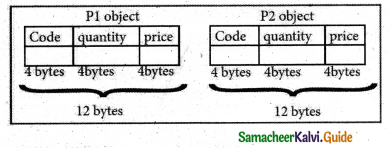Question 5.
Explain the default constructor with an example.
A constructor that accepts no parameter is called the default constructor. For example in the class data program Data::Data() is the default constructor. Using this constructor objects are created similar to the way the variables of other data types are created.

Example:
int num; //ordinary variable declaration
Data d1; // object declarationQuestion 6.
How will you refer members of the class? Give its syntax and an example.
The members of a class are referenced (accessed) by using the object of the class followed by the dot (membership) operator and the name of the member.

The general syntax for calling the member function is:
Object_name.function_name (actual parameter); For example consider the following illustration: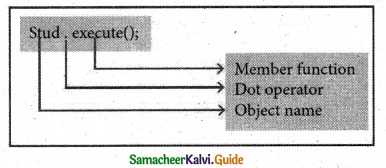Question 7.
Explain the different methods of passing an object to the function argument.
Pass By Value:
When an object is passed by value the function creates its own copy of the object and works on it. Therefore any changes made to the object inside the function do not affect the original object.

Pass By Reference:
When an object is passed by reference, its memory address is passed to the function so the called function works directly on the original object used in the function call. So any changes made to the object inside the function definition are reflected in the original object.

Question 8.
When an instance of a class comes into scope, a special function called the constructor gets executed. The constructor function name has the same name as the class name. The constructors return nothing. They are not associated with any data type. It can be defined either inside class definition or outside the class definition.Question 9.
What is a parameterized constructor?
Parameterized Constructors:
A constructor which can take arguments is called a parameterized constructor. This type of constructor helps to create objects with different initial values. This is achieved by passing parameters to the function.

Example:
class simple
{
private:
int a,b;
public:
simple(int m, int n)
{
a= m ;
b= n;
cout< < “\n Parameterized Constructor of class-simple
}
};

Question 10.
What do you mean by the implicit and explicit call of a constructor?
Implicit call:
In this method, the parameterized constructor is invoked automatically whenever an object is created.
For example, simple s1(10,20); in this for creating the object si parameterized constructor is automatically invoked

Explicit call:
In this method, the name of the constructor is explicitly given to invoking the parameterized constructor so that the object can be created and initialized.

For example:
simple s1=simple(10,20); //explicit callQuestion 11.
When copy constructor Is executed? Give examples.
A copy constructor is called

• When an object is passed as a parameter to any of the member functions
Example: void simple: :putdata(simple x);
• When a member function returns an object
Example: simple get data( ) { }
• When an object is passed by reference to an instance of its own class
For example: simple1, s2(s1); // s2(s1) calls copy constructor

Explain in Detail (5 Marks)

Question 1.
Explain how to define class members?
Definition of class members:
Class comprises of members. Members are classified as Data Members and Member functions.

• Data members are the data variables that represent the features or properties of a class.
• Member functions are the functions that perform specific tasks in a class.
• Member functions are called methods, and data members are also called attributes.

Example: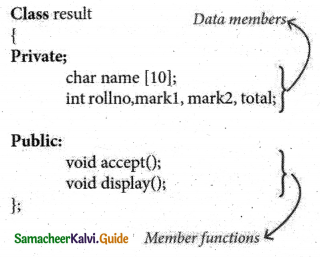Defining methods of a class:
Without defining the methods (functions), class definition will become incomplete. The member functions of a class can be defined in two ways.

• Inside the class definition
• Outside the class definition

Inside the class definition:
When a member function is defined inside a class, it behaves like inline functions. These are called Inline member functions.

Outside the class definition:
When Member function defined outside the class just like normal function definition (Function definitions you are familiar with) then it is being called as an outline member function or non-inline member function. Scope resolution operator (::) is used for this purpose.

The syntax for defining the outline member function is:
return_type class_name :: function name (parameter list)
{
function definition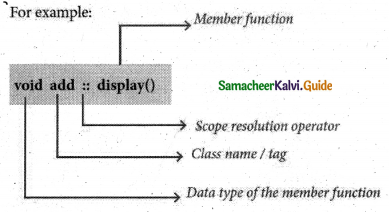Class using Inline and Outline member function:
# include<iostream>
using namespace std;
class Box
{
// no access specifier mentioned
double width;
public:
double length;
//inline member function definition
void printWidth( )
{
cout<<“\n The width of the box is…”<<width;
}
//prototype of the function
void setWidth(double w);
};
// outline member function definition
void Box :: setWidth(double w)
{
width=w;
}
int main( )
{
// object for class Box
Box b;
// Use member function to set the width.
b.setWidth(10.0);
//Use member function to print the width.
b.printWidth( );
return 0;
Output
The width of the box is… 10Question 2.
What are the ways to create an object using the parameterized constructor with an example?
There are two ways to create an object using the parameterized constructor:
1. Implicit call: In this method, the parameterized constructor is invoked automatically whenever an object is created. For example, simple s1( 10,20); in this, for creating the object s1 parameterized constructor is automatically invoked.

2. Explicit call: In this method, the name of the constructor is explicitly given to invoking the parameterized constructor so that the object can be created and initialized.

#include
using namespace std;
class simple
{
private:
int a, b;
public:
simple(int m,int n)
{
a = m;
b = n;
cout << “\n Constructor of class – simple invoked for implicit and explicit call” << endl;
}
void putdata()
{
cout << “\n The two integers are…” << a << ‘\t’ << b << endl;
cout << “\n The sum of the variables” << a << “+” << b << “=” << a + b;
}
};
int main()
{
simple s1(10,20); //implicit call
simple s2 = simple(30,45); //explicit call
cout << “\n\t\tObject 1\n”;
s1.putdata();
s2.putdata();
return 0;
}
Output:
Constructor of class – simple invoked for the implicit and explicit call
Constructor of class-simple invoked for the implicit and explicit call

Object 1
The two integers are… 10 20
The sum of the variables 10 + 20 = 30

Object 2
The two integers are… 30 45.
The sum of the variables 30 + 45 = 75

Question 3.
What are the characteristics of a destructor?
Characteristics of destructors:

• The destructor has the same name as that of the class prefixed by the tilde character
• The destructor cannot have arguments.
• It has no return type.
• Destructors cannot be overloaded i.e., there can be only one destructor in a class.
• In the absence of a user-defined destructor, it is generated by the compiler.
• The destructor is executed automatically when the control reaches the end of the class scope to destroy the object.
• They cannot be inherited.Evaluate Yourself

Question 1.
Define a class in general and in C++’s context.
Classes represent real-world entities that not only have data type properties but also have associated operations.
In C++ class is a way to bind the data and its associated functions together. It is a user-defined data type.

Question 2.
What is the purpose of a class specifier?
Data hiding is one of the important features of Object Oriented Programming which allows preventing the functions of a program to access directly the internal representation of a class type.
The access restriction to the class members is specified by class specifies like public, private, and protected sections within the class body.

Question 3.
Compare a structure and a class in C++ context.
The only difference between structure and class is the members of structure are by default public where as it is private in class.

Question 4.
Compare private and public access specifier.
Public members:
A public member is accessible from anywhere outside the class but within a program. We can set and get the value of public data members even without using any member function.

Private members:
A private member cannot be accessed from outside the class. Only the class member functions can access private members. By default all the members of a class would be private.Question 5.
What is a non-inline member function? Write its syntax.
When Member function defined outside the class just like normal function definition (Function definitions you are familiar with) then it is being called as an outline member function or non-inline member function. Scope resolution operator (::) is used for this purpose.
The syntax for defining the outline member function is:
Syntax:
return_type class_name :: function_name (parameter list)
{
function definition
}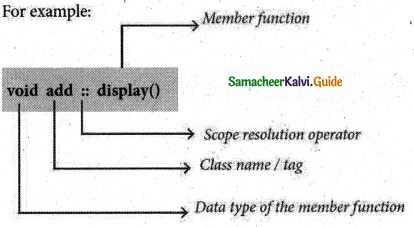Activity – 1
State the reason for the invalidity of the following code fragment.

 (i) (ii) class count { int first int second; public: int first }; class item { int prd; }; int prdno;

• Data member first is duplicated and it is defined with two scopes( both private and public). It is invalid.
• Object name prefix with the only class name. No data type allowed in between class name and object name.Activity – 2
class area
{
int s;
public:
void calc( );
};
Write an outline function definition for calc( ); which finds the area of a square
int area :: calc( ) .
{
return(s * s);
}

Activity – 3
Identify the error in the following code fragment
class A
{
float x;
void init( )
{
Aa1;
X1.5=1; .
}
};
void main( )
{
A1.init( );
}
Error:
Local object can not be accessed from outside the function. Al is the local object, so it can not be accessed in main( );Activity – 4
What is the size of the objects s1, s2?
class sum
{
int n1,n2;
public:
{
int n3=10;n1=n2=10;
}
} s1,s2;
The size of the object SI and S2 is 8 bytes each in Dev C++, In Turbo C++ 4 bytes each.
Program to test the memory requirement:
class sum
{
int n1,n2;
public:
{
int n3-10;
n1=n2=10;
}
} s1,s2;
using namespace std;
#include<iostream>
int main( )
{
cout<<sizeof(s1)<< “”<<sizeof(s2);
}
Output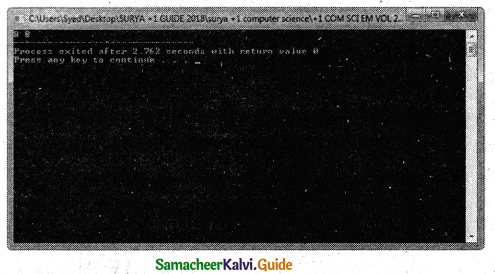Activity – 5
i) Write member function called display with no return.
class objects.
ii) Try the output of the above coding with the necessary modifications.
PROGRAM
#indude<iostream>
using namespace std;
class compute
{
int n1, n2;
public :
void init (int a, int b)
{
n1 = a;
n2 = b;
}
int n;
return (n1+n2);;
{
int prd ( )
{
return (n1*n2);
}
};
compute c1, c2;
void display(compute &objl,compute &obj2)
{
c1.init(12,15);
c2.init(8,4);
cout<<“\n Sum of object-1 “<<obj1.n;
cout<<“\n Sum of object-2 “<<obj2.n;
cout<<“\n Sum of the two objects are”<<obj1.
n+obj2.n;
c1.init(5,4);
c2.init(2,5);
obj1.n = obj1.prd( );
obj2.n = obj2.prd( );
cout<<“\n Product of object-1 “<<objl.n;
cout<<“\n Product of object-2 “<<obj2.n;
cout<<“\n Product of the two objects are “<<objl.n*obj2.n;
}
int main( )
{
display(c1,c2);
return 0;
}
outputActivity – 6
#include<iostream>
using namespace std;
class Sample
{
int i,j;
public :
int k;
Sample( )
{
i=j=k=0;//constructor defined inside the class
}
};
int main( )
{
Sample s1;
return 0;
}
Output
In the above program justify your reason for no output.
Constructor alone is defined without output statement. When the above program is executed, the constructor executed. But no output on the screen because of missing coutHands-On Practice

Question 1.
Define a class employee with the following specification.
private members of class Employee
empno- integer
ename – 20 characters
basic-float
netpay, hra, da, – float
calculate ( ) – A function to find the basic+hra+da with float return type

public member functions of class employee
havedata( ) – A function to accept values for empno, ename, basic, hra,
da and call calculate( ) to compute netpay
dispdata( ) – A function to display all the data members on the screen
PROGRAM
using namespace std;
#include<iostream>
#include<iomanip>
class Employee
{
private :
int empno;
char ename;
float basic,hra,da,netpay;
float calculate( )
{
return (basic+hra+da);
}
public:
void have data( )
{
cout<<setw(35)<<“Enter Employee number :”;
cin>>empno;
cout<<setw(35)<<“Enter Employee name :”;
cin>>ename;
cout<<setw(35)<<“Enter Basic pay :”;
cin>>basic; ,
cout<<setw(35)<<“Enter House Rent Allowance (HRA):”;
cin>>hra; .
cout<<setw(35)<<“Enter Dearness Allowance (DA):”;
cin>>da;
netpay = calculate( );
}
void dispdata( )
{
cout<<“\nEMPLOYEE DETAILS\n\n”;
cout<<setw(35)<<“Employee number :”<<empno<<endl;
cout<<setw(35)<<“Employee name :”<<ename<<endl;
cout<<setw(35)<<“Basic pay :”<<basic<<endl;
cout<<setw(35)<<“House Rent Allowance (HRA) :”<<hra<<endl; cout<<setw(35)<<“Dearness Allowance (DA) :”<<da<<endl;
cout<<setw(35)< <“Netpay :”<<netpay<<endl;
}
};
int main( )
{
Employee e;
e.havedata( );
e.dispdata( );
}
Output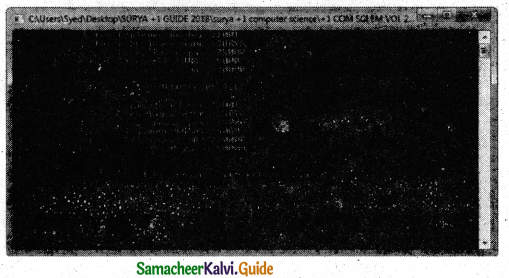Question 2.
Define a class MATH with the following specifications.
private members:
num1, num2, result – float
init( ) function to initialize num1, num2 and result to zero .

protected members:
add( ) function to add num1 and num2 and store the sum in result
diff( ) function to subtract num1 from num2 and store the difference in the result

public members:
getdata( ) function to accept values for num1 and num2
2. Subtract…
invoke add() when the choice is 1 and invoke prod when the choice is 2 and also display the result.
PROGRAM
using namespace std;
#include<iostream>
#include<iomanip>
class MATH
{
private:
float num1,num2,result;
init( )
{
num1=0;
num2=0;
result=0;
}
{
result = num1+num2;
}
void diff( )
{
result = num1 – num2;
}
public:
getdata( )
{
cout<<“\nEntertwo numbers “;
cin>>num1>>num2;
{
int choice;
cout<<“\n2.Subtract …….”;
switch(choice)
{
case 1: getdata( );
break;
case 2: getdata( );
diff( );
cout<<“\nSubtracted value is “<<result;
break;
default: cout<<“\End”;
}
}
};
int main( )
{
MATH m;
}
Output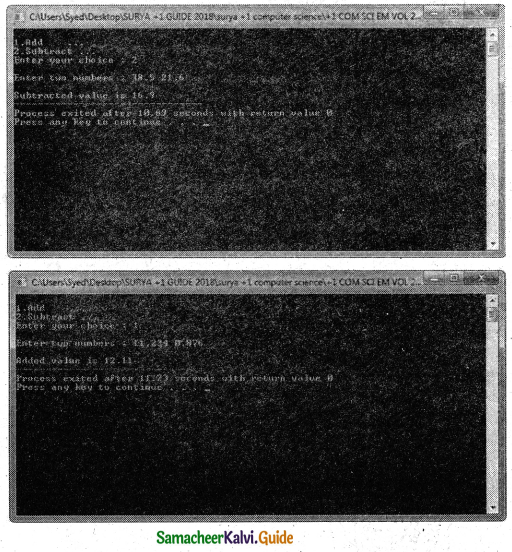Question 3.
Create a class called Item with the following specifications.
private members:
code, quantity- Integer data type
price – Float data type
getdata( )-function to accept values for all , data members with no return

public members:
taxt – float
dispdata( ) member function to display code,quantity,price and tax .The tax is calculated as if the quantity is more than 100 tax is 2500 otherwise 1000.
PROGRAM
using namespace std;
#include<iostream>
#include<iomanip>
class Item
{
private:
int code,quantity;
float price;
void getdata( )
{
cout<<“\nEnter product code “; cin>>code;
cout<<“\nEnter quantity “; cin>>quantity;
cout<<“\nEnter price “; cin>> price;
}
public:
float tax;
void display( )
{
getdata( );
if(quantity>100)
tax = 2500;
else
tax = 1000;
cout<<endl<<setw(25)<< “Product code : “<<code<<endl<<endl;
cout<<setw(25)<<“Quantity : ” <<quantity<<endl<<endl;
cout<<setw(25)<<“Unit price :” <<price<<endl<<endl;
cout<<setw(25)<<“Total Amount: ” < cout<<setw(25)<<“Net Bill amount : ”
<<quantity* price+tax<<endl<<endl;
}
};
int main( )
{
Item i; i.display( );
}
Output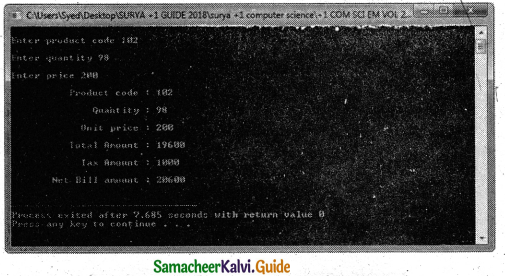Question 4.
Write the definition of a class FRAME in C++ with the following description.
Private members:
FramelD – Integer data type
Height, Width, Amount – Float data type
SetAmount( ) -Member function to calculate and assign amount as 10*Height*Width

Public members:
GetDetail( ) Afunction to allow user to entervalues of FramelD, Height, Width. This function should also call SetAmount() to calculate the amount.
ShowDetail( ) A function to display the values of all data members.
PROGRAM
using namespace std;
#include<iostream>
#include<iomanip>
class FRAME
{
private:
int FrameId;
float Height, Width, Amount;
void SetAmount()
{
Amount = 10 * Height * Width;
}
public:
void Getdetails( )
{
cout<<“\nEnter Frame Id : “; cin>> FrameId;
cout<<“\nEnter Frame Height: cin>> Height;
cout<<“\nEnter Frame Width : “; cin>>Width;
SetAmount( );
}
void ShowDetaiis( )
{
cout<<endl<setw(25)<<“Frame Id :” <<FrameId<<endl<<endl; cout<<setw(25)<<“Frame Height:” <<Height<<endl<endl;
cout<<setw(25)<<“Frame Width :”<<Width<<endk<endl;
cout<<setw(25)<<“Total Amount:”
<<Amount< <endl< <endl;
}
int main( )
{
FRAME F;
F.Getdetails( );
F.ShowDetails( );
}
Output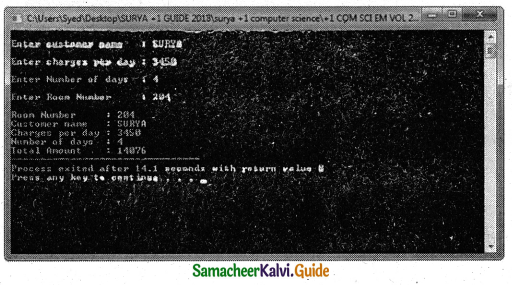Question 5.
Define a class RESORT in C++ with the following description:
Private Members:
Rno //Data member to store Room No
RName //Data member to store customer name
Charges //Data member to store per day charges Days //Data member to store a number of days of stay
COMPUTE( ) //A function to calculate and return Amount as
//Days*Chagres and if the value of Days*Charges is more than 5000 then as 1.02*Days*Charges

Public Members:
Getinfo( ) //A function to enter the content Rno, Name, Charges //and Days Displayinfo( ) //A function to display Rno, RName, Charges, Days and
// Amount (Amount to displayed by calling function COMPUTE())
PROGRAM
using namespace std;
#include<iostream>
class RESORT
{
private:
int Rno,Days,Charges;
char Rname;
int compute( )
{
if (Days * Charges >5000)
return (Days * Charges * 1.02);
else
return(Days * Charges);
}
public:
getinfo( )
{
cout<<“\nEnter customer name :”;
cin>>Rname;
cout<<“\nEnter charges per day :”;
cin>>Charges;
cout<<“\nEnter Number of days :”;
cin>>Days;
cout<<“\nEnter Room Number :”;
cin>>Rno;
}
dispinfo( )
{
cout<<“\nRoom Number : “<<Rno;
cout<<“\nCustomer name :
“<<Rname;
cout«”\nCharges per day :
“<<Charges;
cout<<“\nNumber of days :
“<<Days;
cout<<“\nTotal Amount :
“<<compute( );
}
};
int main( )
{
RESORT Obj;
Obj.getinfo( );
Obj.dispinfo( );
}
Output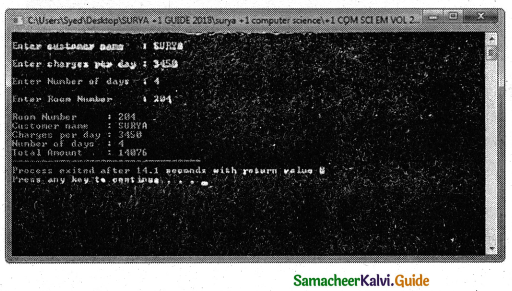Question 7.
struct pno
{
int pin;
float balance;
}
Create a BankAccount class with the following specifications

protected members
pno_obj //array of 10 elements
init(pin) // to accept the pin number and initialize it and initialize
// the balance amount is 0

public members
deposit(pin, amount):
Increment the account balance by accepting the amount and pin. Check the pin number for matching. If it matches increment the balance and display the balance else display an appropriate message withdraw(self, pin, amount):
Decrement the account balance by accepting the amount and pin. Check the pin number for matching and balance is greater than 1000 and amount is less than the balance. If it matches withdraw the amount and display the balance else display an appropriate message
PROGRAM
using namespace std;
#include<iostream>
#include<iomanip>
struct pno
{
int pin;
float balance;
};
class BankAccount
{
public:
pno pno_obj;
void deposit(int pn,float amt)
{
for(int i=0;i<10;i++)
if(pno_obj[i].pin == pn)
{
pno_obj[i].balance = pno_obj[i].balance + amt;
cout<<“\nTransaction successful!
cout<<“\nBalance amount in your account is”<< pno_obj[i].balance; break;
}
void withdraw(int self,int pn,float amt)
{
for(int i=0;i<10;i++)
{
if(pno_obj[i].pin== pn)
{
if (pno_obj[i].balance>1000 && amt < pno_obj[i].balance)
{
pno_obj[i].balance=pno_obj[i]. balance- amt;
cout<<“\nTransaction successful”;
cout<<“\nBalance amount in your account is “<< pno_obj[i].balance; break;
}
} }
}
};
int main( )
{
int pin_no, tamt;
BankAccount b;
// initialization of objects with pin and balance amount as 0
for(int i=0;i<10;i++)
{
b.pno_obj[i].pin=i+l;
}
int choice;
while(choice !=3)
{
cout <<“\n1. Deposit”;
cout <<“\n2.Withdrawal”;
cout<<“\n3.Exit”;
cout<<“\nEnter your choice “; cin >>choice;
switch(choice)
{
case 1:
cout<<“\nEnter PIN
cin>>pin_no;
cout<<“\nEnter Deposit amount”;
cin>>tamt;
b.deposit(pin_no,tamt); break;

case 2:
cout<<“\nEnter PIN cin>>pin_no;
cout<<“\nEnter Withdrawal amount”;
cin>>tamt;
cout<<“\nEnter 1 for Self 2 for Others :”;
int type;
cin>>type;
b.withdraw(type,pin_no,tamt); break;
default: cout<<“\nTransaction completed”;
}
}
}
Output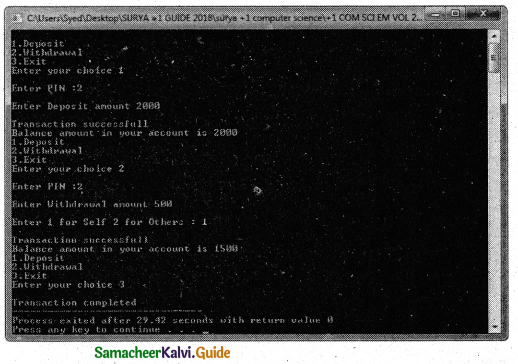Question 8.
Define a class Hotel in C++ with the following description:
Private Members:
Rno //Data member to store Room No
Name //Data member t store customer name
Charges //Data member to store per day charges
Days //Data member to store number of days of stay
Calculate() //A function to calculate and return Amount as
//Days*Chagres and if the value of Days*Charges is more than 12000 then as 1.2*Days*Charges

Public Members:
Hotel( ) //to initialize the class members
Getinfo( ) //A function to enter the content Rno, Name, Charges //and Days
Showinfo( ) //A function to display Rno, RName, Charges, Days and
//Amount (Amount to displayed by calling function CALCULATE( ))
PROGRAM
using namespace std;
#include<iostream>
#include<string.h>
class Hotel
private:
int Rno,Days,Charges;
char Name;
1 int Calculate( )
{
if (Days * Charges >12000)
return (Days * Charges * 1.02);
else
return(Days * Charges);
}
public:
Hotel( )
{
Rno=0;
Days=0;
Charges=0;
strcpy(Name,””);
}
void Getinfo( )
{
cout<<“nEnter customer name :”;
cin>>Name;
cout<<“nEnter charges per day : “;
cin>>Charges;
cout<<“\nEnter Number of days :”;
cin>>Days;
cout<<“\nEnter Room Number :”;
cin>>Rno;
}
void Showinfo( )
{
cout<<“\nRoom Number: “<<Rno;
cout<<“\nCustomer name : “<<Name;
cout<<“\nCharges per day : “<<Charges;
cout<<“\nNumber of days : “<<Days;
cout<<“\nTota! Amount: “<<Calculate( );
}
};
int main( )
{
Hotel obj;
obj.Getinfo( );
obj.Showinfo( )
}
Output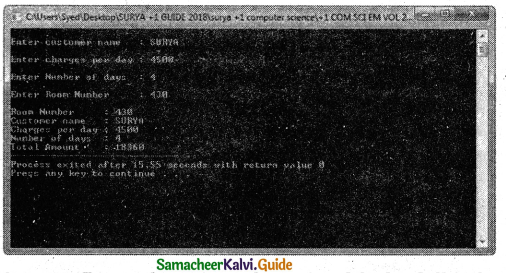Question 9.
Define a class Exam in C++ with the following description:
Private Members:
Rollno – Integer data type
Cname – 25 characters
Mark – Integer data type

public:
Exam(int,char[],int) //to initialize the object ~Exam() // display message “Result will be intimated shortly”
void Display( ) // to display all the details if the mark is above 60 other wise display “Result Withheld”
PROGRAM
using namespace std;
#include<iostream>
#include<string.h>
class Exam
{
private:
int Rollno,Mark;
char Cname;
public:
Exam(int r,char n,int m)
{
Rollno = r;
Mark = m;
strcpy(Cname,n);
}
~Exam( )
{
cout<<“\n\nResult will be intimated shortly”;
}
void Display( )
{
if (Mark>60)
{
cout<<“\n\nRoll Number : “<<Rollno;
cout<<“\nCandidate name : “<<Cname;
cout<<“\nMark :”<<Mark;
}
else
{
cout<<“\n\nRoll Number : “<<Rollno;
cout<<“\nCandidate name : “<<Cname;
cout<<“\nResult Withheld”;
}
}
};
int main( )
{
Exam obj 1(1011,”SURYA”,78),obj2( 1012,”JOHN”,44);
objl.Display( );
obj2. Display( );
}
Output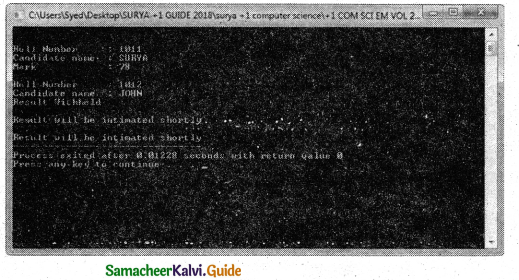Question 10.
Define a class Student in C++ with the following specification:
Private Members:
A data member Rno(Registration Number) type long
A data member Cname of type string A data member Agg_marks (Aggregate Marks) of type float
A data member Grade of type char
A member function setGrade () to find the grade as per the aggregate marks obtained by the student. Equivalent aggregate marks range and the respective grade as shown below.
>=90 – A
Less than 90 and >=75 – B
Less than 75 and >=50 – C
Less than 50 – D
Public members:
A constructor to assign default values to data members:
A copy constructor to store the value in another object
Rno=0, Cname=”N.A” Agg_marks=0,0
A function Getdata ( ) to allow users to enter values for Rno.Cname, Aggjnarks and call functionsetGrade ( ) to find the grade.
A function dispResult( ) to allow user to view the content of all the data members.
A destructor to display the message “END”
PROGRAM .
using namespace std;
#include<iostream>
#include<string.h>
#include<iomanip>
class Student
{
private:
long Rno;
float Agg_marks;
{
if (Ag g_ma rks >=90)
else if(Agg_marks>=75)
else if(Agg_marks>=50)
else
}
public:
Student( )
{
Rno = 0;
Agg_marks = 0;
strcpy(Cname,””);
}
Student(Student &s) .
{
Rno = s.Rno;
Agg_marks = s.Agg_marks;
strcpy(Cname,s.Cname);

~Student( )
{
cout<<“\nEND”; >
void Getdata( )
{
cout<<“\nEnter Register Number”;
cin>>Rno;
cout<<“\nEnter Candidate Name
cin>>Cname;
cout<<“\nEnter Aggrigate Mark”;
cin>>Agg_marks;
}
void dispResult( )
{
cout<<setw(30)<<“Candidate Register Number
“<<Rno<<endl<<endl;
cout< <setw(30)<< “Candidate Name : “<<Cname<<endk<endI;
cout<<setw(30)<<“Aggrigate Mark : “<<Agg_marks«endk<endl;
}
};
int main( )
{
Student s1;
s1.Getdata( );
Student s2(s1);
COut<<“\nFIRST CANDIDATE DETAIL \n\n”; s1.dispResult( );
cout<<“\nSECOND CANDIDATE DETAIL \n\n”;
s2.dispResult( );
}
Output